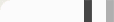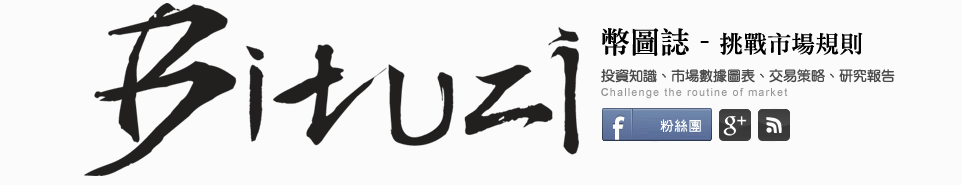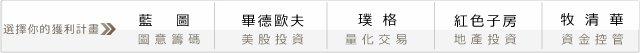## 第一次程式交易就上手－我變我變我變變變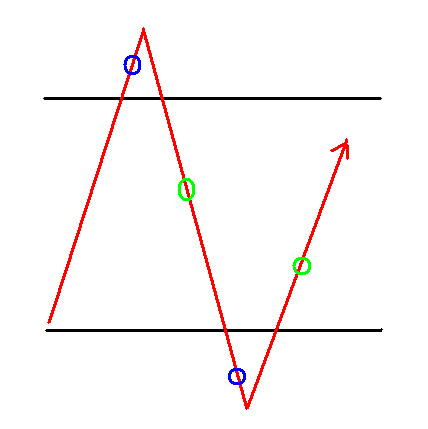#### 回測時間，SHOW TIME!

(1) 當價格往上突破9點前的最高點往上0.5%，我們就進場作多。
(2) 當價格往上突破9點前的最低點往下0.5%，我們就進場作空。

(1) 當價格往上突破9點前的最低點往上1%，我們就進場作多。
(2) 當價格往上突破9點前的最高點往下1%，我們就進場作空。

(1) 設定停損點數為50點。
(2) 1點40分 還有部位就全部出場。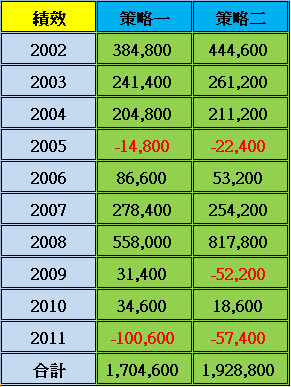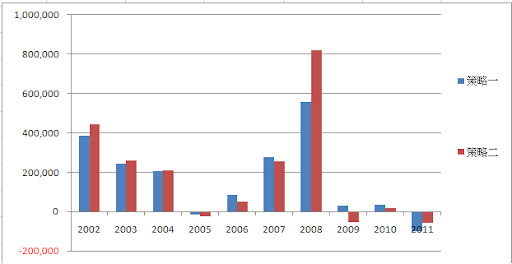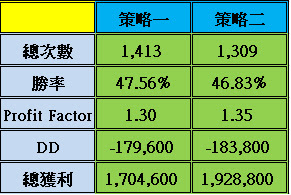input : R(0.01),BeginTime(0900),EndTime(1245);

var :TH(0),TL(0),mkp(0),ax(0),ay(0);

if date <> date then begin
mkp=0;
ax=0;
ay=0;
end;
if time=BeginTime then begin
TH=highd(0);
TL=lowd(0);
end;

if BeginTime < Time and Time < EndTime then begin
if MarketPosition = 0 and ax < 1 and high > TL*(1+R) then buy at highest(h,1)+1 stop;
if MarketPosition = 0 and ay < 1 and low < TH*(1-R) then sell at lowest(l,1)-1 stop;
end;

mkp=marketposition;

if mkp <> 1 and mkp=1 then ax=ax+1;

if mkp <> -1 and mkp=-1 then ay=ay+1;

if marketposition =1 then begin
exitlong at entryprice-50 stop ;
if time>1339 then exitlong this bar on close;
end;

if marketposition =-1 then begin
exitshort at entryprice+50 stop ;
if time>1339 then exitshort this bar on close;
end;

setexitonclose;

#### 0 意見: